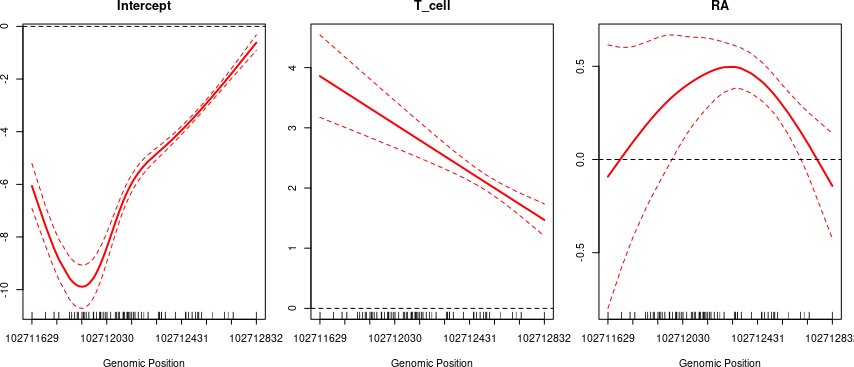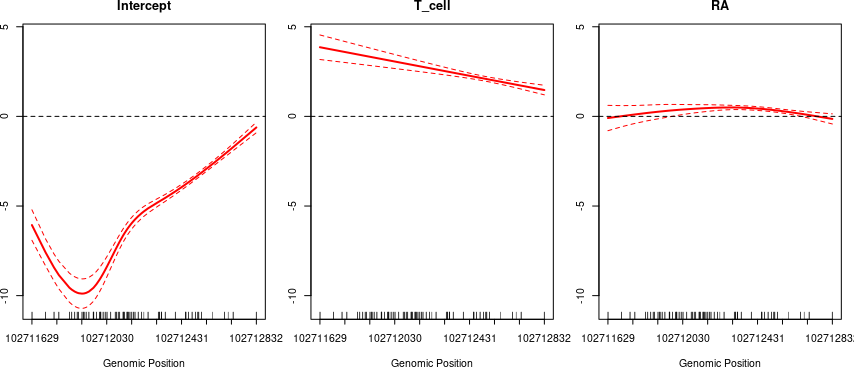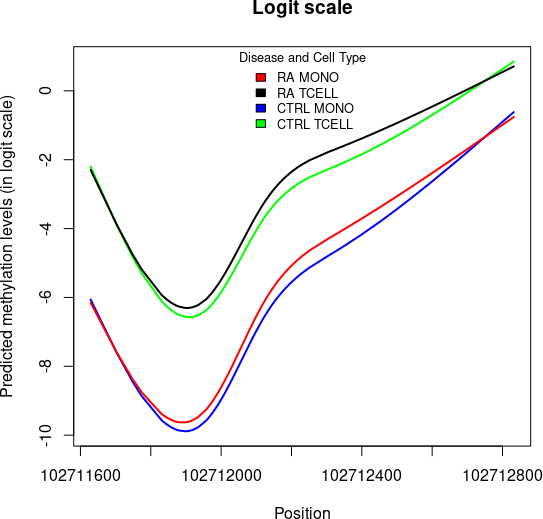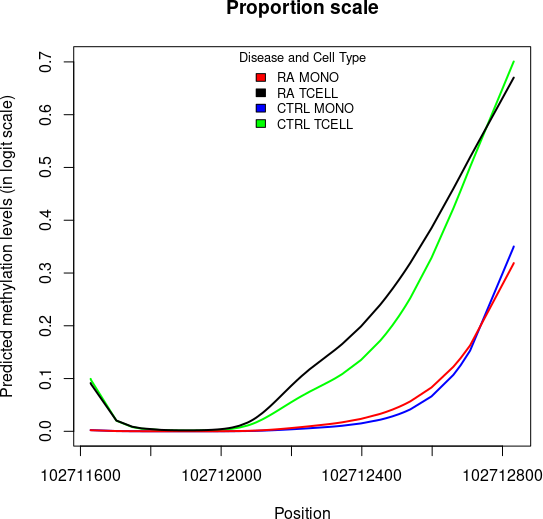# 1 Installation

Install the package SOMNiBUS from Bioconductor.

if (!requireNamespace("BiocManager", quietly=TRUE))
install.packages("BiocManager")

BiocManager::install("SOMNiBUS")

# 2 Introduction

SOMNiBUS aims to analyze count-based methylation data on predefined genomic regions, such as those obtained by targeted sequencing, and thus to identify differentially methylated regions (DMRs) that are associated with phenotypes or traits surch as cell types.

• enable complex associations with multiple phenotypes / traits using a Generalized Additive Model approach
• the modeling strategy incorporates count-based methylation error rate arguments (i.e., p0 or false positive rate and p1 or true positive rate)

For a more comprehensive introduction of the SOMNiBUS approach, please read our SOMNiBUS paper (Kaiqiong Zhao 2020).

## 2.1 Citation

If you use this package, please cite our SOMNiBUS paper (Kaiqiong Zhao 2020).

# 3 Application

## 3.1 Rheumatoid arthritis study

Throughout this vignette, we illustrate the SOMNiBUS approach with analysis of a targeted region from a rheumatoid arthritis (RA) study. See help(RAdat) for further details. In this example, the phenotype of major interest is the RA status (coded as RA) and the adjusting variable is the cell type status (coded as T_cell) which is binary because the experiment used cell-type-separated blood samples, and methylation profiles were characterized for both T-cells and Monocytes. We will refer to both RA and T_cell as covariates. We are going to use the package SOMNiBUS to investigate the methylation patterns in this region and study association with RA status and cell type.

library(SOMNiBUS)

# 4 Input data

Currently, we require a matrix-type input of the methylated reads (Meth_Counts) and the read depth (Total_Counts) for CpG sites of each sample. Inputs in another format, such as Bismark or a BSeq object from the bsseq package, will be incorporated in the future.

Before using the package, the input data matrix (or data frame) should be formatted such that:

1. each row represents a CpG site
2. the first 4 columns should contain the information of Meth_Counts (methylated counts), Total_Counts (read depths), Position (Genomic position for the CpG site) and ID (sample ID)
3. the covariate(s), such as disease status or cell type composition are listed in column 5 and onwards.

An example of the input data:

head(RAdat)
#>   Meth_Counts Total_Counts  Position ID T_cell RA
#> 1           0            8 102711629  1      0  1
#> 2           0            2 102711630  1      0  1
#> 3           0           12 102711702  1      0  1
#> 4           0            4 102711703  1      0  1
#> 5           0           15 102711747  1      0  1
#> 6           0            6 102711748  1      0  1

## 4.1 Filtering CpGs and samples

To better use the information in the methylation dataset, on one hand, SOMNiBUS uses a smoothing technique (regression splines) to borrow information from the nearby CpG sites; on the other hand, our approach uses regression-based modelling to take advantage of information contained across samples. Therefore, this algorithm does not require filtering out the CpG sites that have methylation levels measured only in a small part of the samples, or the samples that have overall poor read-depths and many missing values. Our analysis of differentially methylated regions (DMRs) requires filtering only on the following two conditions:

• individual CpGs that have zero reads in a particular sample (no observation available)
• samples that have missing values in any of the covariates of interest (i.e missing values for T_cell or RA in the data set RAdat)
RAdat.f <- na.omit(RAdat[RAdat$Total_Counts != 0, ]) ## 4.2 Adjusting for covariates and adding interactions • we currently only accept numeric input for the covariates used to fit the model. we recommend that first you transform your categorical variables into appropriate dummy variables • interaction terms can be added in the analysis model. To do that, the program requires that users add another column of covariate values into the input data set calculated as the product of two existing covariates whose interaction is of interest. # 5 Analysis The smooth covariate estimation and the region-wise test steps are wrapped into a function binomRegMethModel. See help(binomRegMethModel) for more details. We can use the following code to run the analysis with both covariates T_cell and RA. out <- binomRegMethModel(data = RAdat.f, n.k = rep(5, 3), p0 = 0.003, p1 = 0.9, Quasi = FALSE, RanEff = FALSE) Or, we can use the argument covs to specify that we only want the covariate T_cell in the model. out.ctype <- binomRegMethModel(data = RAdat.f, n.k = rep(5, 3), p0 = 0.003, p1 = 0.9, covs = "T_cell") ## 5.1 Error rates p0 and p1 In the example data set, we have cell type separated samples. The error rates for individual samples can be estimated by a E-M algorithm (Lakhal-Chaieb et al. 2017) using the package SmoothMSC. The error rate default values, $$p_0=0.003$$ and $$p_1=0.9$$, were estimated as the average incomplete ($$p_0$$) or over- conversion ($$1-p_1$$) of the metabisulfite. These two estimated values coincide roughly with the incomplete and over conversion rates related to bisulfite sequencing experiment reported in Prochenka et al. (2015). Both parameters, p0 and p1, correspond to the false positive rate and the true positive rate respectively, where 1-p1 being the false negative rate. For experiments with samples from a tissue containing a mixture of cell types, the user could consider the following ways to specify the error rates p0 and 1-p1. • If users have conducted experiments for measuring error/conversion rates, such as adding spike-in sequences of DNA that are known in advance to be methylated or unmethylated into the bisulfite sequencing procedure, they can use the measured error rates for the input of p0 and p1 • One can also use the error rates (incomplete and over conversion rates) that have been previous reported in the literature. • Another option is to use our default values. ## 5.2 Basis dimensions n.k: Argument n.k in the binomRegMethModel is the dimension of the basis expansion for smooth covariate effects. The exact number n.k used for each functional parameter is not crucial, because it only sets an upper bound. We recommend choosing a basis dimension approximately equal to the number of unique CpGs in the region divided by 20. as.integer(length(unique(RAdat.f$Position)) / 20)
#>  6

# 6 Results

## 6.1 testing the null hypothesis

Under the null hypothesis, we are expecting no effects of the covariates over the region-wide methylation status.

out$reg.out #> EDF Chi.sq p-value #> Intercept 3.999034 584.06810 0 #> T_cell 2.007277 999.31851 0 #> RA 3.903903 80.44909 0 ## 6.2 Estimation of the smooth covariate effects binomRegMethModelPlot(out)Figure 1: The estimates (solid red lines) and 95% pointwise confidence intervals (dashed red lines) of the intercept, the smooth effect of cell type and RA on methylation levels We can also force the covariate effect plots to have the same vertical range, for all covariates, by specifying same.range= T. binomRegMethModelPlot(out, same.range = TRUE)Figure 2: The estimates (solid red lines) and 95% pointwise confidence intervals (dashed red lines) of the intercept, the smooth effect of cell type and RA on methylation levels (Same ranges of Y axis.) ## 6.3 Predicted methylation levels First, construct a new data set for prediction. Make sure that the Position in the new data set is the same as the original input data in binomRegMethModel. pos <- out$uni.pos
my.p <- length(pos)
newdata <- expand.grid(pos, c(0, 1), c(0, 1))
colnames(newdata) <- c("Position", "T_cell", "RA")

The predicted methylation levels can be calculated from function binomRegMethModelPred

my.pred <- binomRegMethModelPred(out, newdata, type = "link.scale")
plot(pos[order(pos)], (my.pred[(newdata$RA == 0 & newdata$T_cell == 0)])[order(pos)],
type = "l", xlab = "Position",
ylab = "Predicted methylation levels (in logit scale)", col = "blue", main = "Logit scale", ylim = c(min(my.pred), max(my.pred)), lwd = 2
)
lines(pos[order(pos)], (my.pred[(newdata$RA == 0 & newdata$T_cell == 1)])[order(pos)],
type = "l", xlab = "Position",
ylab = "predicted", col = "green", lwd = 2
)
lines(pos[order(pos)], (my.pred[(newdata$RA == 1 & newdata$T_cell == 0)])[order(pos)],
type = "l", xlab = "Position",
ylab = "predicted", col = "red", lwd = 2
)
lines(pos[order(pos)], (my.pred[(newdata$RA == 1 & newdata$T_cell == 1)])[order(pos)],
type = "l", xlab = "Position",
ylab = "predicted", col = "black", lwd = 2
)
legend("top", c("RA MONO", "RA TCELL", "CTRL MONO", "CTRL TCELL"),
fill = c("red", "black", "blue", "green"),
title = "Disease and Cell Type", bty = "n", cex = 0.8
)Figure 3: The predicted methylation levels in the logit scale for the 4 groups of samples with different disease and cell type status

my.pred <- binomRegMethModelPred(out, newdata, type = "proportion")
plot(pos[order(pos)], (my.pred[(newdata$RA == 0 & newdata$T_cell == 0)])[order(pos)],
type = "l", xlab = "Position",
ylab = "Predicted methylation levels (in logit scale)", col = "blue", main = "Proportion scale", ylim = c(min(my.pred), max(my.pred)), lwd = 2
)
lines(pos[order(pos)], (my.pred[(newdata$RA == 0 & newdata$T_cell == 1)])[order(pos)],
type = "l", xlab = "Position",
ylab = "predicted", col = "green", lwd = 2
)
lines(pos[order(pos)], (my.pred[(newdata$RA == 1 & newdata$T_cell == 0)])[order(pos)],
type = "l", xlab = "Position",
ylab = "predicted", col = "red", lwd = 2
)
lines(pos[order(pos)], (my.pred[(newdata$RA == 1 & newdata$T_cell == 1)])[order(pos)],
type = "l", xlab = "Position",
ylab = "predicted", col = "black", lwd = 2
)
legend("top", c("RA MONO", "RA TCELL", "CTRL MONO", "CTRL TCELL"),
fill = c("red", "black", "blue", "green"),
title = "Disease and Cell Type", bty = "n", cex = 0.8
)Figure 4: The predicted methylation levels proportion scale (right) for the 4 groups of samples with different disease and cell type status

# Session info

Here is the output of sessionInfo() on the system on which this document was compiled running pandoc 2.9.2.1:

#> R Under development (unstable) (2022-10-25 r83175)
#> Platform: x86_64-pc-linux-gnu (64-bit)
#> Running under: Ubuntu 22.04.1 LTS
#>
#> Matrix products: default
#> BLAS:   /home/biocbuild/bbs-3.17-bioc/R/lib/libRblas.so
#> LAPACK: /usr/lib/x86_64-linux-gnu/lapack/liblapack.so.3.10.0
#>
#> locale:
#>   LC_CTYPE=en_US.UTF-8       LC_NUMERIC=C
#>   LC_TIME=en_GB              LC_COLLATE=C
#>   LC_MONETARY=en_US.UTF-8    LC_MESSAGES=en_US.UTF-8
#>   LC_PAPER=en_US.UTF-8       LC_NAME=C
#>  LC_MEASUREMENT=en_US.UTF-8 LC_IDENTIFICATION=C
#>
#> attached base packages:
#>  stats     graphics  grDevices utils     datasets  methods   base
#>
#> other attached packages:
#>  SOMNiBUS_1.7.0   BiocStyle_2.27.0
#>
#> loaded via a namespace (and not attached):
#>   nlme_3.1-160        cli_3.4.1           knitr_1.40
#>   magick_2.7.3        rlang_1.0.6         xfun_0.34
#>   highr_0.9           stringi_1.7.8       jsonlite_1.8.3
#>  htmltools_0.5.3     stats4_4.3.0        sass_0.4.2
#>  rmarkdown_2.17      grid_4.3.0          evaluate_0.17
#>  jquerylib_0.1.4     fastmap_1.1.0       yaml_2.3.6
#>  bookdown_0.29       BiocManager_1.30.19 stringr_1.4.1
#>  compiler_4.3.0      Rcpp_1.0.9          VGAM_1.1-7
#>  mgcv_1.8-41         lattice_0.20-45     digest_0.6.30
#>  R6_2.5.1            splines_4.3.0       magrittr_2.0.3
#>  Matrix_1.5-1        bslib_0.4.0         tools_4.3.0
#>  cachem_1.0.6